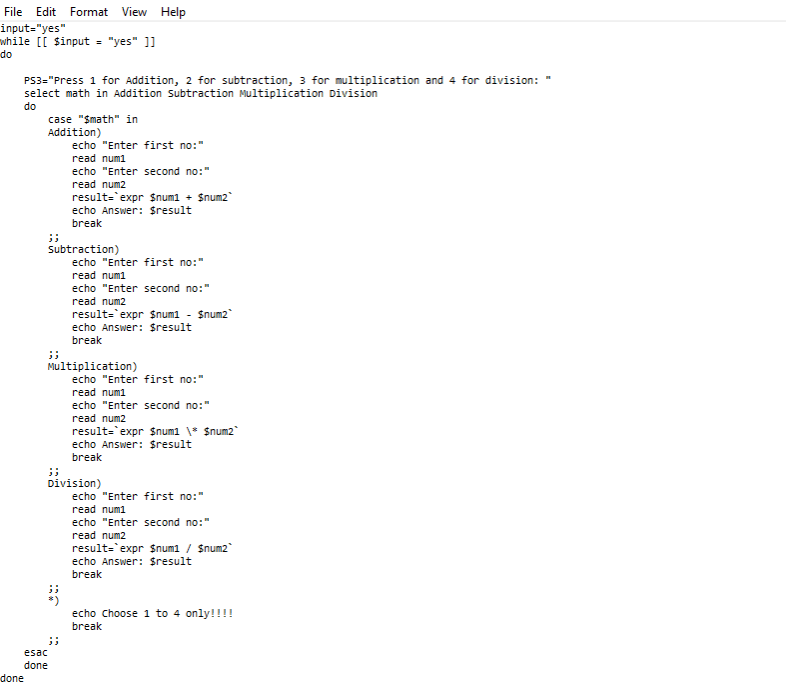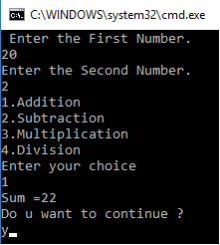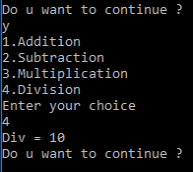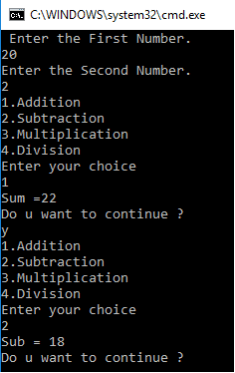System ProgrammingLearn To Create A Shell Script Calculator using Switch Case

# Learn To Create A Shell Script Calculator using Switch Case

In this blog, we will learn how to create a simple calculator that will be able to perform arithmetic operations like addition, subtraction, multiplication, and division. This operation will depend on the user input in a Bash shell.

Let’s first take a look at a couple of Bash commands that can help you become a little more familiar with the project before we start coding the calculator.

Commands / Statement Used:

1. Echo
echo is one of the most used commands. It is used to print a line of text in the standard output\$ echo [-neE] [arg …]

The read command in the Linux is used to read the input from the keyboard.

3. Switch-Case
When there are a lot of “if” statements in Shell, it becomes confusing. There, it is a good option to use a case statement.

The switch command can only be used in batch files. It allows you to select a command or group of commands to execute based on the possible values of a variable or a combination of variables and text.

The SWITCH command is always followed by an expression created from environment variables, internal variables, variable functions, and text strings, and then by a sequence of CASE statements matching the possible values of expression, an optional DEFAULT statement, and terminated by an ENDSWITCH statement. Each CASE statement and the DEFAULT statement may be followed by one or more commands.

4. Basic Commands (BC) – BC commands are used in a Command Line Calculator to perform basic Mathmatical Calculations.

```Syntax: bc [ -hlwsqv ] [long-options] [ file ... ]
Options:
-h, {- -help }: Print the usage and exit
-i, {- -interactive }: Force interactive mode.
-q, {- -quiet }:  Do not print the normal GNU bc welcome.
-v, {- -version }: Prints the version number, copyright info and quits.```

The bc command supports the following features:
• Arithmetic operators
• Increment or Decrement operators
• Assignment operators
• Comparison or Relational operators
• Logical or Boolean operators
• Math functions
• Conditional statements
• Iterative statements

Example –
\$ echo “15+5” | bc
Output: 20

5. While –
The bash while loop is a control flow statement that allows code commands to be executed repeatedly based on a given condition.

I can use this following syntax to perform math operations.
\$((expression)) or \$[expression],
let “expression” or ((expression)).

In the first case, an expression is evaluated and interpreted as a command or a parameter. In the second, an expression is evaluated and a return value \$? is set to false (1) if its evaluated value is zero and or true (0) if it is non-zero. We can use variables with or without a prefix dollar symbol, so the syntax \$((x))and \$((\$x)) will return the same value if \$x contains a valid numeric value.

ash works only with integers, its data type is signed int. My 64bit Fedora installation has a range from –263 to 263–1. Numbers are stored in two’s complement format, so in hexadecimal notation, it is from 0x8000000000000000 to 0x7fffffffffffffff, so beware of number overflow.In this command prompt, you can see the codes display an addition of two numbers. The user can enter a first and second number and it will display the sum of both the numbers.In this command prompt, you can display a subtraction of two numbers. The user can enter a first and second number and it will display the result after subtraction.This command prompt will display a multiplication of two numbers. The user can enter two numbers and it will show you the result of multiplied numbers.
This command prompt will display a division of two numbers. The user can enter two numbers and it will show you the result of divided numbers.Conclusion– Hope this has been helpful for you guys to learn how to create your own Shell Script Menu Driven Calculator using Switch Case.

### Build A Front End Of an e-Commerce Site Using React

21,501Fans
4,213Followers
104,000Subscribers

### Build A Front End Of an e-Commerce Site Using React

- Advertisement -